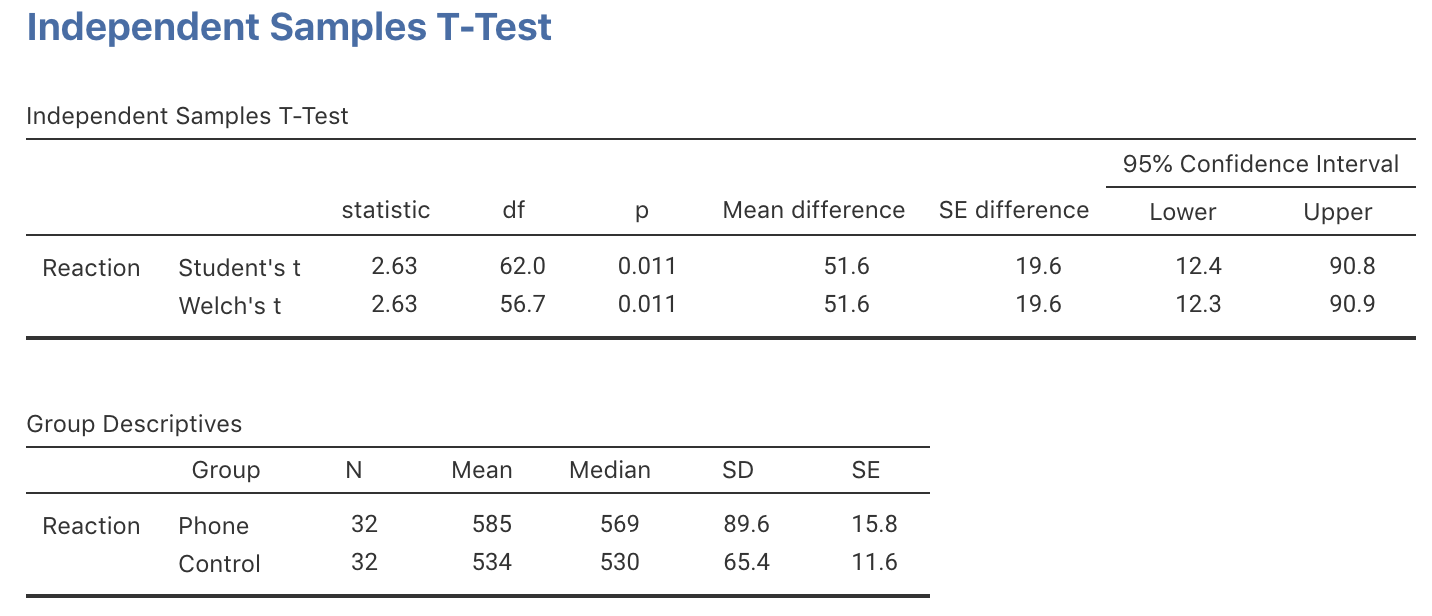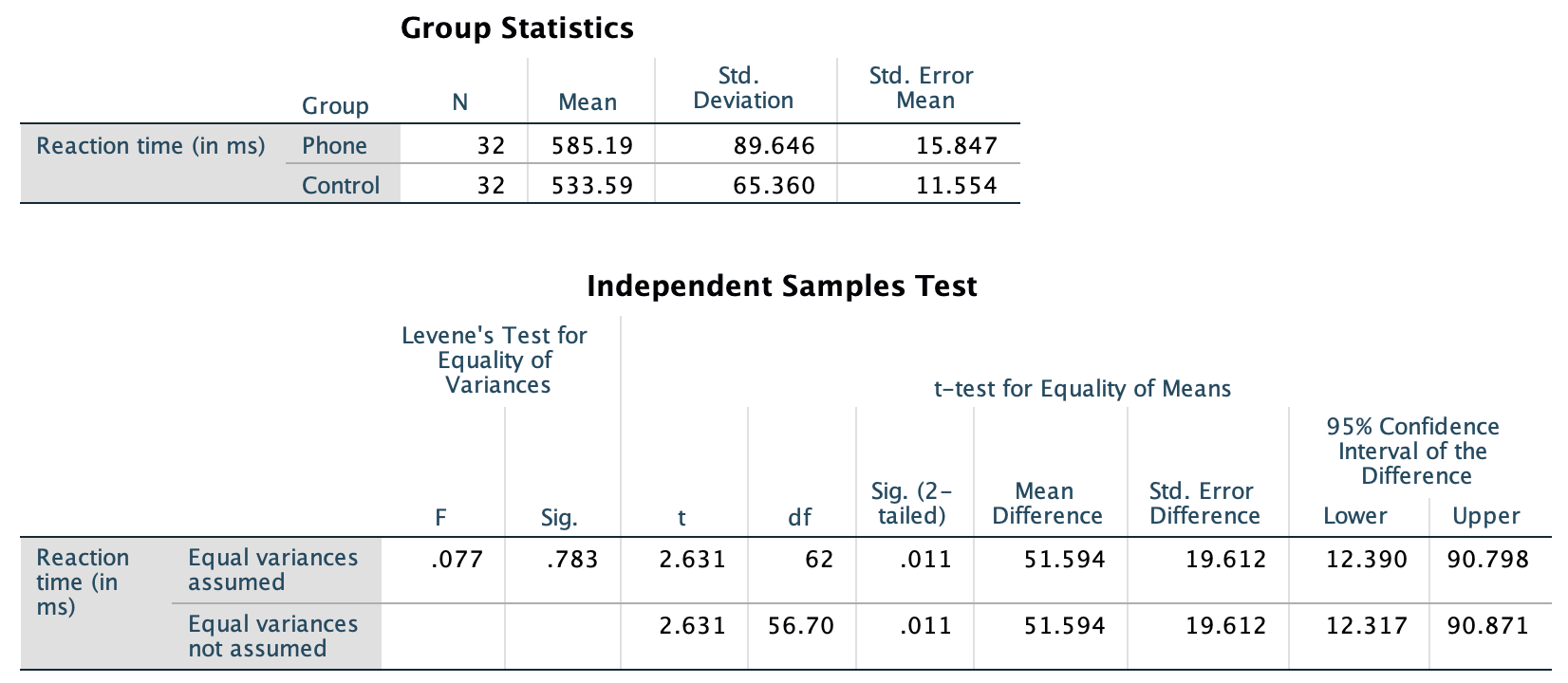## 30.3 Sampling distribution: Two independent means

The data for testing the hypothesis are shown below. The numerical summary (Sect. 24.1) must summarise the difference between the means (since the RQ is about the difference), and should summarise each group. All this information is found using software (jamovi: Fig. 30.1; SPSS: Fig. 30.2), and can be compiled into a table (Table 30.2).

The appropriate summary for graphically summarising the data is a boxplot (though a dot chart is also acceptable). An error bar chart (Fig. 24.7), which allows the sample means to be compared, should also be produced.FIGURE 30.1: jamovi output for the phone reaction time dataFIGURE 30.2: SPSS output for the phone reaction time data

The difference between the sample means is 51.59 ms… but this value will vary from sample to sample; that is, there is sampling variation. The sampling variation (expectation) for the values of $$\bar{x}_A - \bar{x}_B$$ can be described as having:

• an approximate normal distribution;
• centred around $${\mu_{P}} - {\mu_{C}} = 0$$ (from $$H_0$$);
• with a standard deviation of $$\displaystyle\text{s.e.}(\bar{x}_P - \bar{x}_C)$$, called the standard error for the difference between the means.
jamovi and SPSS give results from two similar hypothesis tests. In this book, we will always use the second row of information (the “Welch’s $$t$$” row in jamovi; the “Equal variance not assumed” row in SPSS) because it is more general and makes fewer assumptions.
TABLE 30.2: Numerical summaries of the reaction-time data (in milliseconds)
Mean Sample size Standard deviation Standard error
Using phone 585.1875 32 89.6460558116231 15.8473334927566
Not using phone 533.59375 32 65.3599756065616 11.5541204923968
All students 51.59375 19.6121309189865